Refer to our Texas Go Math Grade 8 Answer Key Pdf to score good marks in the exams. Test yourself by practicing the problems from Texas Go Math Grade 8 Module 10 Quiz Answer Key.

10.1 Surface Area of Prisms

Find the lateral and total surface area of each prism. Round your answers to the nearest tenth if necessary.

Question 1.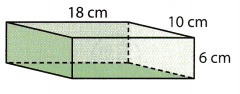Given that the prism is a rectangular prism.
Lateral surface area of a rectangular prism = 2(l + w)h.
Length = l = 18cm
Width = w = 10cm
Height = h = 6cm.
=2(18 + 10)6.
=2(28)6.
= 336 square units.

Question 2.Given that the prism is the triangular prism.
The formula for the lateral surface of the triangular prism = perimeter of the base x height of the prism = (a + b + c)h.
H = 8yd
A = 25yd
B = 10yd
C = 6yd
= (25 + 10 + 6)8
= (41)8
= 328yd.
The lateral surface area of the triangular prism = 328 yd²
328 rounded to the nearest ten is 330. Because in the 336 the one’s place is greater than the 5. Thus replace 0 by 0 and tens place by 3.

Question 3.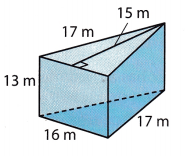Given that the prism is the triangular prism.
The formula for the lateral surface of the triangular prism = perimeter of the base x height of the prism = (a + b + c)h.
H = 15m
A = 17m
B = 13m
C = 16m
= (17 + 13 + 16)15
= (46)15
= 690m.
The lateral surface of the triangular prism = 690m²
690 rounded to the nearest ten is 690.

Question 4.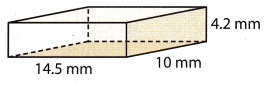Given that the prism is a rectangular prism.
Lateral surface area of a rectangular prism = 2(l + w)h.
Length = l = 14.5mm
Width = w = 10mm
Height = h = 4.2mm
=2(14.5 + 10)4.2.
=2(24.5)4.2.
= 205.8mm²
205.8 rounded to the nearest ten is 205. Because in the 205.8 the decimal place is greater than the 5. So replace the 8 by 0

10.2 Surface Area of Cylinders

Find the lateral and total surface area of each cylinder. Round your answers to the nearest tenth if necessary. Use 3.14 for π.

Question 5.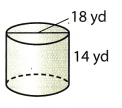Formula for the lateral surface area of the cylinder is 2πRH.
Diameter = 18yd
H = height = 14yd
LA = 2 x 3.14 x 9 x 14.
lateral surface area of the cylinder= 791.28yd.
791.28 is rounded to the nearest tenth is 791.20. Because in the decimal the one’s place is greater than 5 so it is replaced by 0.

Question 6.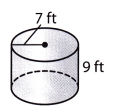The formula for the lateral surface area of the cylinder is 2πRH.
H = height = 9ft
LA = 2 x 3.14 x 7 x 9.
the lateral surface area of the cylinder= 395.64ft
395.64 is rounded to the nearest tenth is 1582.60. Because in the decimal the one’s place is less than 5 so it is replaced by 0.

Essential Question

Question 7.
Answer: The packaging boxes are in the shape of a cuboid and it has 6 faces. Its label has length, width, and height. The surface area of the prism is SA = 2lw + 2lh + 2hw.

Texas Go Math Grade 8 Module 10 Mixed Review Texas Test Prep Answer Key

Selected Response

Question 1.
Grain is stored in cylindrical structures called silos. Which is the best estimate for the volume of a silo with a diameter of 12.3 feet and a height of 25 feet?
(A) 450 cubic feet
(B) 900 cubic feet
(C) 2970 cubic feet
(D) 10,800 cubic feet
The formula for the volume of the cylinder = 2πr2h.
H = 25feet
Diameter = 12.3 feet
Radius = d/2 = 12.3/2 =6.15.
= 2 x 3.14 x (12.3)2 x 25
= 2969.06625 = 2970 cubic feet.
Option C is the correct answer.

Question 2.
What is the side length of a cube that has a total surface area of 384 square inches?
(A) 6 inches
(B) 7 inches
(C) 8 inches
(D) 9 inches
Given that total surface area of the cube = 384 inches
Formula for the total surface area = 6a²
= 6(6)² = 216 inches.
= 6(7)² = 294 inches.
= 6(8)² = 384 inches.
= 6(9)² = 486 inches.
Option C is the correct answer.

Question 3.
A block of cheese is shown in the shape of the triangular prism below.What is the total surface area of the block of cheese?
(A) 120 in2
(B) 494 in2
(C) 510 in2
(D) 640 in2
There are two triangles in the triangular prism
Area of the one triangle = ½ x b x h
= ½ x 17 x 15 = 127.5
Area of the two triangle = ½ x b x h
= 1/2 x 8 x 13 = 52
The formula for the total surface area of the triangle = area of one triangle + area of two triangles.
= 179.5 square inches.

Question 4.
Using 3.14 for π, what is the lateral surface area of the cylinder to the nearest unit?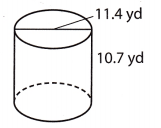(A) 122 yd2
(B) 204 yd2
(C) 383 yd2
(D) 587 yd2
The formula for the lateral surface area of the cylinder is 2πRH.
Diameter = 11.4yd
H = height = 10.7yd
LA = 2 x 3.14 x 5.7 x 10.7.
the lateral surface area of the cylinder= 383.01yd2
383.01 is rounded to the nearest tenth of 383. Because in the decimal the one’s place is less than 5 so it is replaced by 0.
Option C is the correct answer.

Question 5.
The net of a cylinder is shown.
What is the total surface area of the cylinder represented by the net?(A) 1607.7 m2
(B) 1856 m2
(C) 4220.2 m2
(D) 5827.8 m2
The formula for the surface area of the cylinder = 2πr(r + h).
H = 42m
Diameter = 32m
= 2 x 3.14 x 16(16 + 42)
= 100.48(58)
= 5827.8
Option D is the correct answer.

Question 6.
A cylinder has a circumference of 12π cm and a height that is half the radius. What is the total surface area of the cylinder?
(A) 367π cm2
(B) 72π cm2
(C) 108π cm2
(D) 144π cm2
The formula for the surface area of the cylinder = 2πr(r + h).
Circumference = 12π/π
= 12 is the diameter.
Radius = d/2 = 12/2 = 6
R = 6
H = half the radius = 6/2 = 3.
= 2 x π x 6(6 + 3)
= 6.28 x 54
= 108πcm²
Option C is the correct answer.

Gridded Response

Question 7.
A rectangular prism has a length of 4 feet, a width of 1 yard, and a height of 3 inches. What is the total surface area of the prism to the nearest tenth of a square foot?